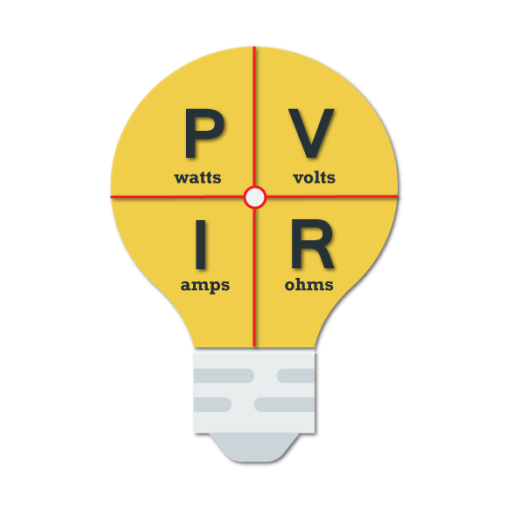#### Current Version : 1.2 Downloads : 34,792 Size : 7.3 MB

Electrical Calculator and Formula is an app that will help you calculate useful Electrical engineering formulas in a very simple way. The app includes has a list of electrical formula to help you recall the method of calculations. The app includes formulas with an appropriate description. The app can be very much helpful in generator sizing calculator for various motor loads.

<b>List of electrical formulas available for calculations are</b>
1) <b>SMD Register Code calculations</b>
2) <b>Wire Size</b> - Here you can calculate wire size for Single as well as Three Phase connection. You can select material like copper or aluminum used in a wire. Here you need to supply Voltage, Load (Ampere, Watt, KiloWatt, Horse Power), Distance (in feet, or in meter), and Power Factor.

3) <b>Ohm’s Law</b> - Calculate Voltage using a combination of
- Current and Resistance
- Power and Resistance
- Power and Current
Similarly calculate values for Current, Resistance, and Power.
4) <b>Series-Parallel</b> - Here calculate values for
- Resister in Series
- Register in Parallel
- Capacitor in Series
- Capacitor in Parallel
- Inductor in Series
- Inductor in Parallel

5) <b>Single Phase</b> - Calculations available here includes
- One Phase Power
- One Phase Voltage
- One Phase Current
- One Phase Power Factor
- One Phase Kva
Formulas available here helps you understand the calculation in a much better way.

6) <b>3 Phase formula with calculations</b>
- 3 Phase Power
- 3 Phase Voltage
- 3 Phase Current
- 3 Phase Power Factor
- 3 Phase KVA

7) <b>Conversion</b> - Available calculations includes
- Star to Delta
- Delta to Star
- HP - KW conversion
- KW to HP conversion
- KW to KVA conversion with KW formula
- KVA to KW conversion with KVA formula
- Electricity Power/Bill Calculator
- Electrical Power Calculator
- Wire Size Conversion Chart

8) <b>Color Code</b> -
- Resistor Color Code
- Here you can calculate resistance for 4 Band, 5 Band, and 6 Band resistance.
- Inductor Color Code
- Here calculation available includes for 4 Band resistance.

-----------------------------------------------------------------------------------------------------------------------

This App is developed at ASWDC by Meet Janani (150540107032), a 5th Sem CE Student. ASWDC is Apps, Software, and Website Development Center @ Darshan Institute of Engineering & Technology, Rajkot run by Students & Staff of Computer Engineering Department

Call us: +91-97277-47317

Write to us: aswdc@darshan.ac.in
Visit: http://www.aswdc.in http://www.darshan.ac.in

ASWDC is established by Department of Computer Engineering where students work on live projects under guidance of staff and industry experts. Students are getting extensive knowledge and industrial experience of cutting edge technologies. ASWDC fills gap between academic curriculum and industry expectation.